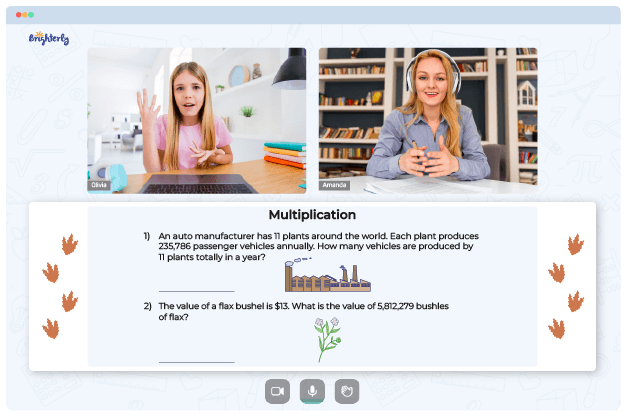# 2 Digit By 2 Digit Multiplication Worksheets

Multiplication is one of the basic operations in math that every kid needs to understand. When dealing with 2-digit by 2-digit multiplications, kids often need all the guidance they can get. But what if you are not around to assist them? 2-digit by 2-digit multiplication worksheets can help, and this article will describe how.

## Why Brighterly’s Tutors Use 2-Digit by 2-Digit Multiplication Worksheets

Educators use two-digit by two-digit multiplication worksheets in various ways, as a means of assessment, practice, and instructional materials. The use of a multiplication 2-digit by 2-digit worksheet to learn in a study group or for peer tutoring can help students improve their skills.

Math for Kids

Is Your Child Struggling With Math?
1:1 Online Math TutoringBrighterly is an online math learning platform with professionals committed to giving kids only the best math education. The tutors provide kids with 2-digit by 2-digit multiplication worksheets as PDF files to simplify complex multiplication. Parents can also use them to help their kids acquire a new math ability or keep them rehashing existing knowledge over the holidays.

## 2-Digit by 2-Digit Multiplication Worksheets Allow Teach Math in a Fun Way### 2 Digit By 2 Digit Multiplication Worksheets PDF

2-Digit By 2-Digit Multiplication Worksheets### 2 Digit By 2 Digit Multiplication Worksheets PDF

2 Digit By 2 Digit Multiplication Worksheets With Grids### 2 Digit By 2 Digit Multiplication Worksheets PDF

Multiplication 2 Digit By 2 Digit Worksheet### 2 Digit By 2 Digit Multiplication Worksheets PDF

Two Digit By Two Digit Multiplication Worksheets

A multiplying by two-digit numbers worksheet comes with math riddles, word problems, brainteasers, and puzzles. 2-digit by 2-digit multiplication worksheets with grids make help elementary school students practice multiplying two-digit numbers by two-digit numbers using the grid technique instead of traditional methods.

Because of the intriguing designs of these worksheets, kids do not need to stress themselves when solving math tasks. They often think of it as an exciting activity, similar to playing a game or achieving a goal.

Helping your kid with online math lessons for 4th grade. Expert tutors inspiring kids to love math so they can excel at It.

### More Multiplication Worksheets

Problems with Multiplication?• Does your child struggle to grasp multiplication concepts?
• Try learning with an online tutor.

Is your child having trouble with understanding multiplication concepts? An online tutor could provide the necessary support.